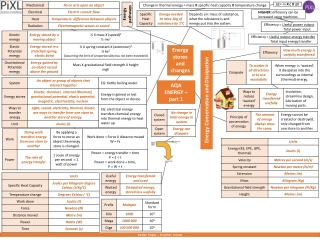DownloadDownload PresentationAQA ENERGY – part 1

# AQA ENERGY – part 1

Download Presentation## AQA ENERGY – part 1

- - - - - - - - - - - - - - - - - - - - - - - - - - - E N D - - - - - - - - - - - - - - - - - - - - - - - - - - -
##### Presentation Transcript

1. Energy pathways Change in thermal energy = mass X specific heat capacity X temperature change ∆E= m X cX ∆θ HIGHER: efficiency can be increased using machines. Efficiency = Useful power output Total power input Efficiency = Useful output energy transfer Total input energy transfer Energy stores and changes Energy Conservation and Dissipation AQA ENERGY – part 1

2. Power station – NB: You need to understand the principle behind generating electricity. An energy resource is burnt to make steam to drive a turbine which drives the generator. Using renewable energy will need to increase to meet demand. Energy demand is increasing as population increases. Renewable energy makes up about 20% of energy consumption. Fossil fuel reserves are running out. AQA ENERGY – part 2 Using fuels Global Energy Resources National Grid Energy resources

3. Electrons carry current. Electrons are free to move in metal. Series and parallel circuits Circuit symbols Current and Charge Current, potential difference and resistance Controlling current Q = I X t Charge = Current X time R = V X I Energy transfers Power (W) = potential difference X current AQA Electricity Work is done when charge flowing. P = I2 X R Power = (current)2 X resistance E = P X t Energy transferred = Power X time R = V ÷ I Domestic uses and safety Resistance = Potential difference ÷ Current ‘Earthing’ a safety device; Earth wire joins the metal case. Current – Potential difference graphs

4. Pressure of a fixed volume of gas increases as temperature increases (temperature increases, speed increases, collisions occur more frequently and with more force so pressure increases). Temperature of gas is linked to the average kinetic energy of the particles. Kinetic theory of gases Particle model If kinetic energy increases so does the temperature of gas. No kinetic energy is lost when gas particles collide with each other or the container. AQA PARTICLE MODEL OF MATTER Gas particles are in a constant state of random motion. Change in thermal energy = mass X specific heat capacity X temperature change. P = m ÷ V Density = mass ÷ volume. ∆E= m X cX ∆θ Internal energy and energy transfers Change of state Energy needed = mass X specific latent heat. ∆E= m X L

5. Radius of an atom 1 X 10-10m Electrons gained Electrons lost Negative ion Positive ion Atom structure Atoms and Nuclear Radiation Atoms and Isotopes AQA ATOMIC STRUCTURE PHYSICS ONLY: Hazards and uses of Radioactive emissions and of background radiaton Discovery of the nucleus

6. Each Kg has a gravitational pull of 9.8N. The component forces combined have the same effect. Weight = mass X gravitational field strength W = m X g HIGHER ONLY Gravity Work done against frictional forces, temperature of object rises. Object moves left with a force of 5N Contact and Resultant forces Forces and their interactions If force is at right angles to direction of movement, NO work is done. AQA FORCES – part 1 Work done and energy transfer Scalar and vector quantities Forces and elasticity Pressure HIGHER ONLY

7. Transverse and Longitudinal waves Waves in air, fluids and solids In water, use a ripple tank. Properties Measuring speed In air, use echoes. AQA Waves e.g. Gamma Short wavelengths have high frequency and high energy. Electromagnetic waves HIGHER: Properties Absorbed light changes into thermal energy store. Seismic waves EM waves refract

8. Magnetic fields from the permanent magnet and current in the foil interact. This is called the motor effect. Reverse current, magnetic field direction reverses. AQA MAGNETISM AND ELECTROMAGNETISM Reverse the current , foil moves upwards. Further away from the wire, magnetic field is weaker. Aluminium foil placed between two poles of a strong magnet, will move downwards when current flows through the foil. Current large enough, iron filings show circular magnetic field. Size of force acting on foil depends on magnetic flux density between poles, size of current, length of foil between poles. Electric current flowing in a wire produces a magnetic field around it. If current is small, magnetic field is very weak. Permanent and Induced Magnetism Magnets